# 1.- Introduction

The Egg of Life is the name given to the second iteration in the process of construction of the Flower of Life. Therefore it contains 7+6=13 circles as shown in Figure 1. This figure surely can be translated to three dimensions in several ways. Here we propose two of them.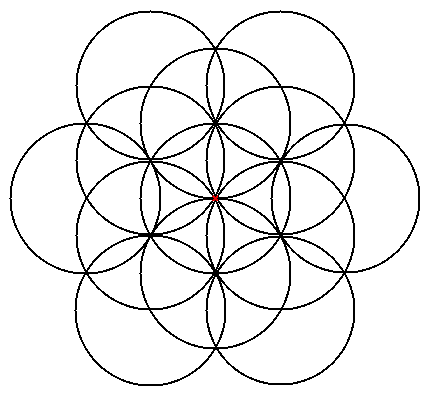Figure 1: The Egg of Life contained in the pattern of the Flower of Life.

# 2.- The Egg of Life as a Vector Equilibrium

There is a three dimensional structure contained inside Metatron's Cube grid that contains exactly 13 vertices: the central cuboctahedron, or actually a vector equilibrium (see Figure 3 below). If one draws a sphere centered in each of its vertices such that all the spheres are tangent to each other, the resulting figure has a two dimensional projection identical to that of the Egg of Life, as shown in Figure 2: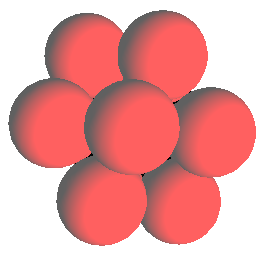Figure 2: The three dimensional Egg of Life as the vertices of a cuboctahedron with a central vertex, the so called vector equilibrium.

Although this 3D structure coincides both in projection and in number of spheres with its corresponding 2D counterpart, we have found nobody that talks about it. One point against it would be the fact that its spheres are not contained among the spheres of the 3D Metatron's Cube. The next structure proposed solves this drawback.

# 3.- The Egg of Life as a Star Tetrahedron

Taking the preceding cuboctahedron as the starting grid, we know that it contains eigth tetrahedrons inside, plus six half octahedrons. The following figure shows the XY coordinates of the cuboctahedron.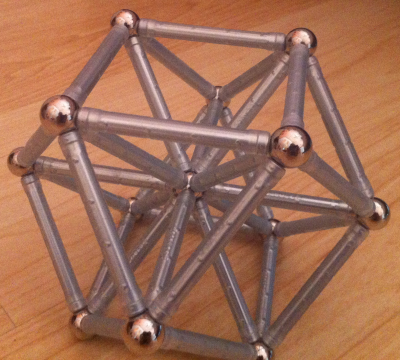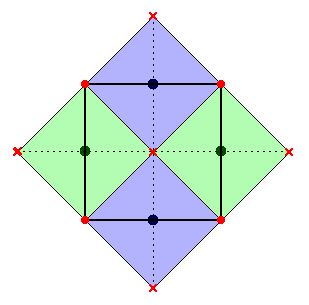(a) XY projection of the cuboctahedron grid with a central vertex (vector equilibrium). (b) A cuboctahedron grid with a central vertex (vector equilibrium) (c) The four tetrahedrons in the upper layer. The layer below contains the remaining four, oriented in the orthogonal direction. Figure 3

We can draw a sphere in the center of each tetrahedron and join them forming of a cube. But we can also join them in an alternative way, forming a couple of tetrahedrons intersecting as a star tetrahedron of spheres. This is what we tried to illustrate with two different colors in Figure 3c and in Figure 4. The eight inner tetrahedrons actually contain the eight central spheres of Metatron's Cube. The following figure illustrates this structure using the pattern contained in the Flower of Life: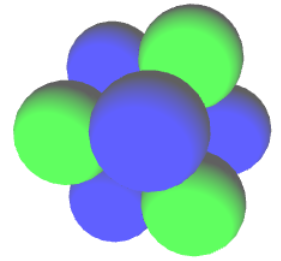(a) Eight spheres in the Egg of Life forming the vertices of two tetrahedrons joined as a star tetrahedron or a cube. (c) Three dimensional view of the Fruit of Life according to its structure as eight spheres. Click on the image to get a 3D model that you can zoom, rotate and pan. (b) The Flower of Life pattern also shows that the eight spheres in the Egg of Life are contained in a cuboctahedron. Figure 4

This is the structure of the Egg of Life proposed by Drunvalo Melchizedek in the first volume of his book "The ancient secret of the Flower of Life". It has the drawback that the number of spheres (8) does not coincide with the number of circles in the 2D pattern (13). But it has the advantage that it is formed by eight spheres contained in the center of 3D Metatron's Cube. Drunvalo also affirms in his book that this structure is adopted by our first eight cells in the body. Those cells are located in our exact geometric center, and they are the only ones that never die to be regenerated.